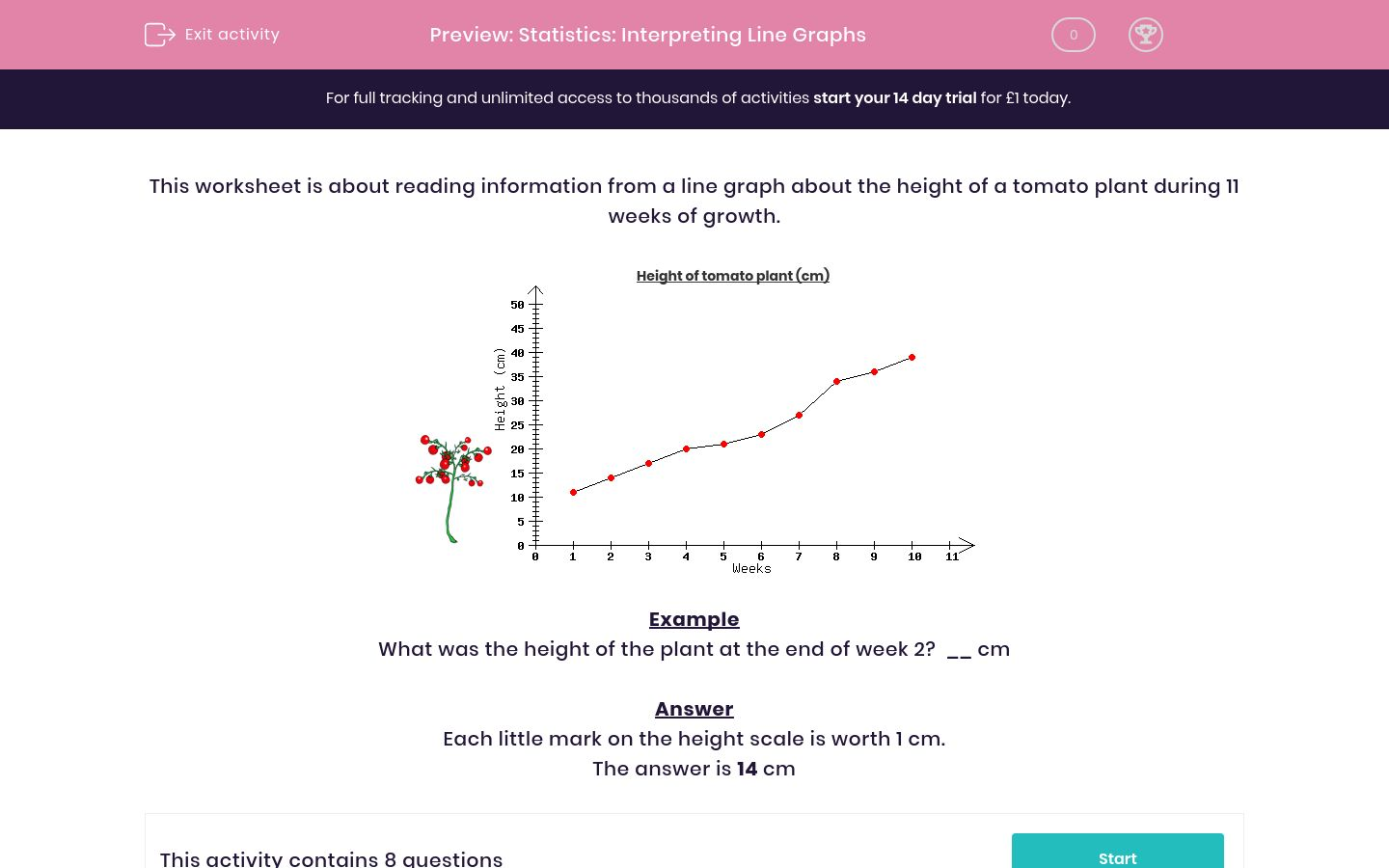# Statistics: Interpreting Line GraphsKey stage:  KS 2

Curriculum topic:   Maths and Numerical Reasoning

Curriculum subtopic:   Interpreting Data

Difficulty level:### QUESTION 1 of 10

This worksheet is about reading information from a line graph about the height of a tomato plant during 11 weeks of growth.Height of tomato plant (cm)Example

What was the height of the plant at the end of week 2?  __ cm

Each little mark on the height scale is worth 1 cm.

Answer the question related to the line graph.Height of tomato plant (cm).What was the height of the plant at the end of week 10?  __ cm

Answer the question related to the line graph.Height of tomato plant (cm)How many centimetres did the plant grow between weeks 6 and 7? __ cm

Answer the question related to the line graph.Height of tomato plant (cm)Between which two weeks did the plant grow fastest?

Between weeks 7 & 8

Between weeks 10 & 11

Answer the question related to the line graph.Height of tomato plant (cm)Estimate the plant's height halfway between weeks 3 and 4.    __ cm

Answer the question related to the line graph.Height of tomato plant (cm)What was the height of the plant at the end of the first week?  __ cm

Answer the question related to the line graph.Height of tomato plant (cm)How many centimetres did the plant grow between weeks 1 and 2? __ cm

Answer the question related to the line graph.Height of tomato plant (cm)Between which two weeks did the plant grow the fastest?

Between weeks 7 & 8

Between weeks 6 & 7

Answer the question related to the line graph.Height of tomato plant (cm)Estimate the plant's height at week 5? __ cm

• Question 1

Answer the question related to the line graph.Height of tomato plant (cm).What was the height of the plant at the end of week 10?  __ cm

39
40
• Question 2

Answer the question related to the line graph.Height of tomato plant (cm)How many centimetres did the plant grow between weeks 6 and 7? __ cm

4
5
• Question 3

Answer the question related to the line graph.Height of tomato plant (cm)Between which two weeks did the plant grow fastest?

Between weeks 7 & 8
• Question 4

Answer the question related to the line graph.Height of tomato plant (cm)Estimate the plant's height halfway between weeks 3 and 4.    __ cm

18
19
• Question 5

Answer the question related to the line graph.Height of tomato plant (cm)What was the height of the plant at the end of the first week?  __ cm

6
• Question 6

Answer the question related to the line graph.Height of tomato plant (cm)How many centimetres did the plant grow between weeks 1 and 2? __ cm

1
• Question 7

Answer the question related to the line graph.Height of tomato plant (cm)Between which two weeks did the plant grow the fastest?

Between weeks 6 & 7
• Question 8

Answer the question related to the line graph.Height of tomato plant (cm)Estimate the plant's height at week 5? __ cm

10
---- OR ----

Sign up for a £1 trial so you can track and measure your child's progress on this activity.

### What is EdPlace?

We're your National Curriculum aligned online education content provider helping each child succeed in English, maths and science from year 1 to GCSE. With an EdPlace account you’ll be able to track and measure progress, helping each child achieve their best. We build confidence and attainment by personalising each child’s learning at a level that suits them.

Get started